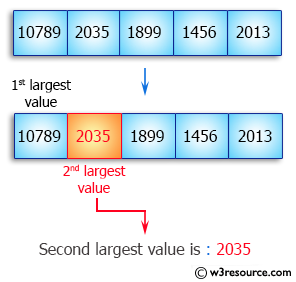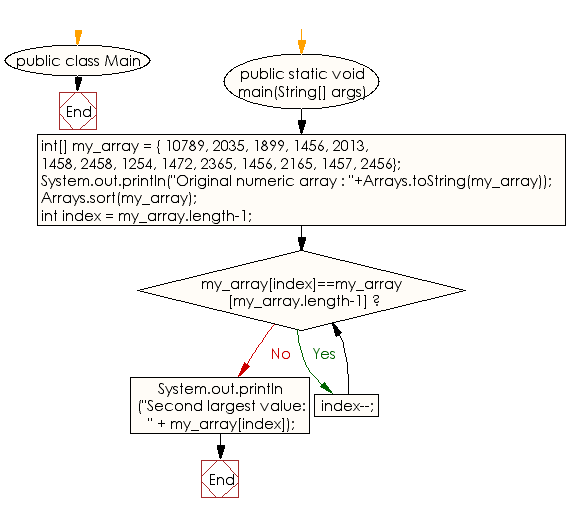﻿ Java exercises: Find the second largest element in an array - w3resource# Java Array Exercises: Find the second largest element in an array

## Java Array: Exercise-17 with Solution

Write a Java program to find the second largest element in an array.

Pictorial Presentation:Sample Solution:

Java Code :

``````import java.util.Arrays;
public class Main {
public static void main(String[] args) {
int[] my_array = {
10789, 2035, 1899, 1456, 2013,
1458, 2458, 1254, 1472, 2365,
1456, 2165, 1457, 2456};
System.out.println("Original numeric array : "+Arrays.toString(my_array));
Arrays.sort(my_array);
int index = my_array.length-1;
while(my_array[index]==my_array[my_array.length-1]){
index--;
}
System.out.println("Second largest value: " + my_array[index]);
}
}
```
```

Sample Output:

```Original numeric array : [10789, 2035, 1899, 1456, 2013, 1458, 2458, 1254, 1472, 2365, 1456, 2165, 1457, 2456]
Second largest value: 2458
```

Flowchart:Visualize Java code execution (Python Tutor):

Java Code Editor:

Improve this sample solution and post your code through Disqus

What is the difficulty level of this exercise?

Test your Programming skills with w3resource's quiz.

﻿

## Java: Tips of the Day

countOccurrences

Counts the occurrences of a value in an array.

Use Arrays.stream().filter().count() to count total number of values that equals the specified value.

```public static long countOccurrences(int[] numbers, int value) {
return Arrays.stream(numbers)
.filter(number -> number == value)
.count();
}
```

Ref: https://bit.ly/3kCAgLb